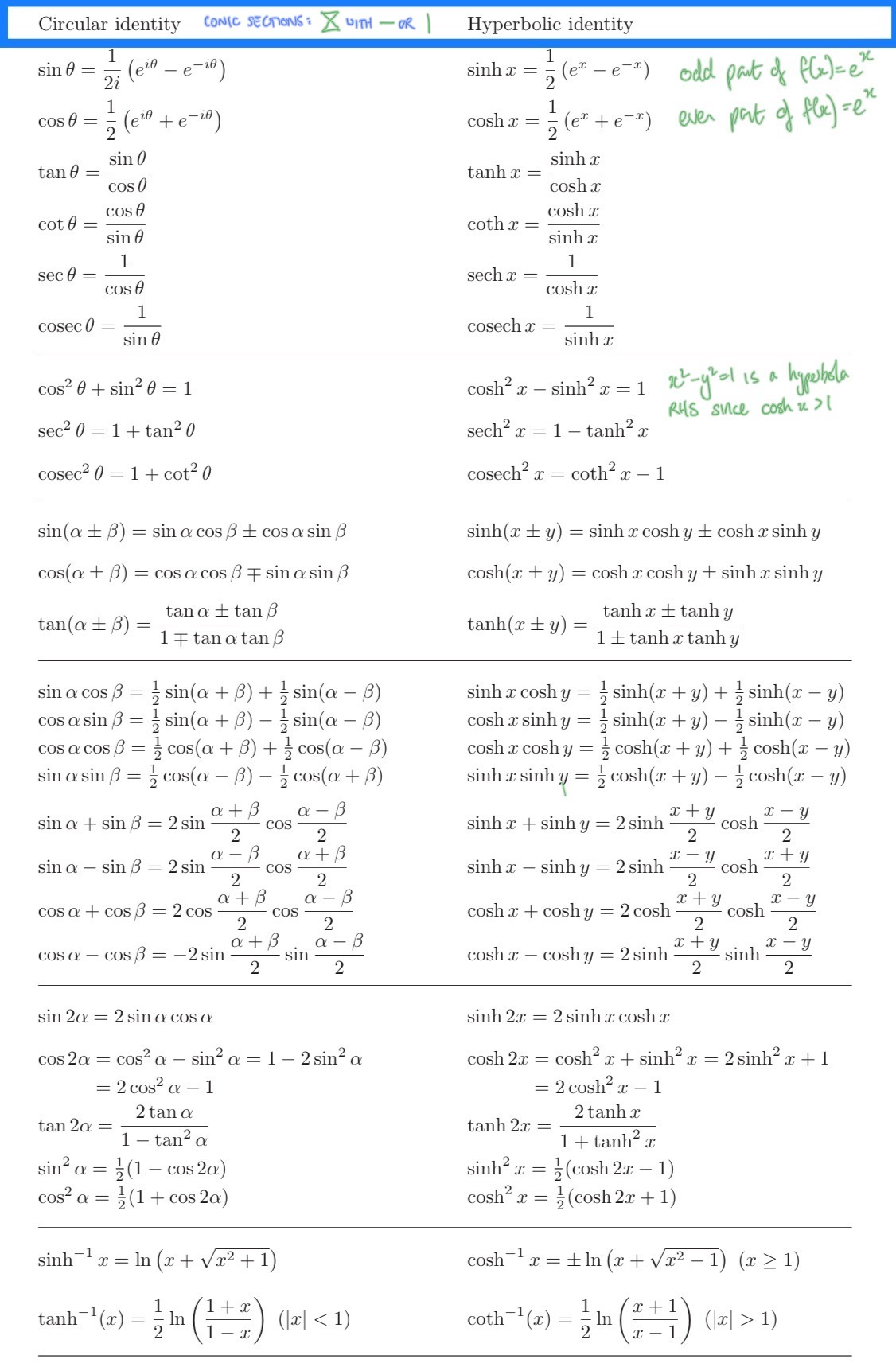## 5.1 Identities

### 5.1.1 Trigonometric

[]

### 5.1.2 Antiderivatives

Simple functions, nb relationship to gradient and derivative is zero at max/min of the function.

Table 5.1: Antiderivatives of Common Functions

(a) Common Functions
$$\int f(x)\, dx$$ $$f(x)$$
$$x^n$$ $$nx^{n-1}$$
$$\exp{g(x)}$$ $$g'(x) \exp{g(x)}$$
$$\log g(x)$$ $${g'(x)}/{g(x)}$$
$$\tan^{-1}(x)$$ $$\frac{1}{1+x^2}$$
$$\sin^{-1}(x)$$ $$\frac{1}{\sqrt{1-x^2}}$$
(b) Trigonometric
$$\int f(x)\, dx$$ $$f(x)$$
$$\sin (x)$$ $$\cos (x)$$
$$\cos (x)$$ $$-\sin (x)$$
$$\sec^2 (x)$$ $$\tan (x)$$
$$\sec(x)\tan(x)$$ $$\sec(x)$$
$$\csc^2(x)$$ $$-\cot(x)$$
$$\csc(x)\cot(x)$$ $$-\csc(x)$$

## 5.2 Simple Formulae

• arithmetic series
if $$u_n = a + (n-1)d$$ then $$S_n = \frac{1}{2}n[2a+(n-1)d]$$ (cf Gauss)
• geometric series
if $$u_n = ar^{n-1}$$ then $$S_n = \frac{a}{1-r} \quad |r|<1$$ (cf $$S-xS=a$$)
• binomial expansion
$$(a+b)^n = \binom{n}{1} a^n + \binom{n}{2} a^{n-1}b + \dots$$ with
$$\binom{n}{k} = \frac{n!}{k!(n-k)!}$$
• quadratic formula (cf Vieta’s formulae)
$$\frac{-b \pm \sqrt{b^2-4ac}}{2a}$$
• Newton-Raphson
$$x_{n+1}=x_n - \frac{f(x_n)}{f'(x_n)}$$

### 5.2.1 Bounds

• Markov Inequality
• AM/GM Inequality
• Cauchy-Schwartz Inequality
• Triangle Inequality
• Cramer-Rao Lower Bound